Next: Text in Math Mode Up: Commands for Math Mode Previous: Numbered Equations

## Subscripting and Superscripting

A subscript is indicated by the underscore _''. For example,

 $\alpha_j$ gives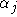$\alpha^j$ gives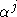$\alpha_j^i$ gives$\alpha_{j^i}$ givesThis brings us to a point about using the curly brackets {}. They are used a lot in math mode to group things together. Many math commands in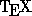are defined to operate on the next item which appears in the file: either a single letter, a command word, or a group delimitted by braces. For instance, the subscripting operator _'' can be used to subscript a single letter ($a_b$) or a single command ($a_\beta$). But if you want a subscript containing something more complicated, say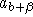, then you must use braces: $a_{b+\beta}$.

Another example of when the curly braces must be used is if you want the subscripts lower and smaller. Then, you would type

$\alpha_{_j}$.

This applies the lowering operator twice, to yield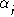.

The expression $\alpha__j$ will generate an error message.

Processed by LaTeX2html